Theory.org » fracdyn » burningship » Julia Sets from the Burning Ship

Julia Sets from the Burning Ship

Beginning with the quadratic Burning Ship, z -> (|re(z)|+i*|im(z)|)2+c, we examine some Julia sets. The large image below of the Burning Ship shows where the Julias are taken from. Note that +y is down, not up. This is the standard in computer graphics, and BFE just happened to get written that way.

The Julias taken from along the real axis are identical to Julias taken from the z -> z2 Mandelbrot. This happens because if y=0, z2 = x2 = |x|2.

The Burning Ship and the Mandelbrot do not share Julias for any other poins where y!=0. This is because the orbits of all points will eventually take a point to somewhere in the second or fourth quadrants (-x,y) or (x,-y). The y value of the next iteration from within one of these quadrants will be i*2*|-x|*|y| or i*2*|x|*|-y|. Neither of these are the same as the y-value for a point iterated through the Mandelbrot, i*2*x*y, because of the absolute value operation .

To show that all points will pass through this condition, all we need to show is that the coordinates will eventually have opposite sign. If a point is already in one of these quadrants, our condition is satisfied. If not, we have three conditions.

First, if x2 = y2, then our series goes as follows:
(x, y)
(x2-y2, 2*|x|*|y|) = (0, 2*|x|*|y|)
(0-(2*|x|*|y|)2, 2*0*2*|x|*|y|) = (-(2*|x|*|y|)2, 0) = (-x, 0)

Second, if x2 < y2,then our series goes as follows:
(x, y)
(x2-y2, 2*|x|*|y|) = (-x, y)

Third, if x2 > y2,then our series goes as follows:
(x, y)
(x2-y2, 2*|x|*|y|) = (x, y)
((x2-y2)2-4*x2*y2, 2*2*|x|*|y|*(x2-y2) )
Which simplifies to:
(x4-6*x2*y2+y4, 4*|x|3*|y|-4*|y|3*|x|)
By looking deeper in to the iterations, you can continually eliminate smaller and smaller chunks of the fractal. I believe that there is probably some inductive way to show that the rest of the julias are different than the Mandelbrot set's.

Burning Ship Home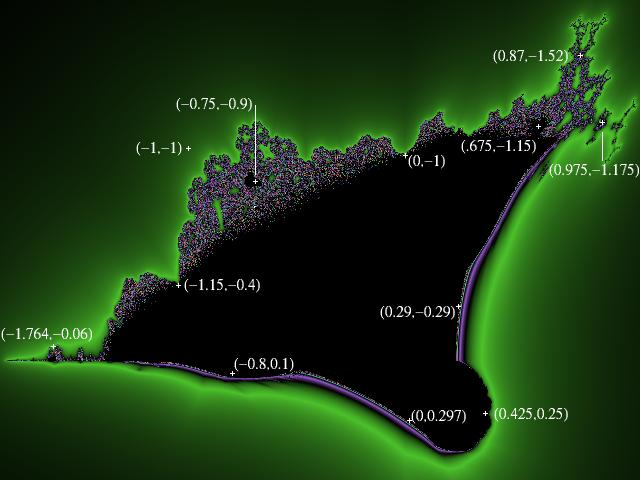The Julia sets are arranged clockwise from left.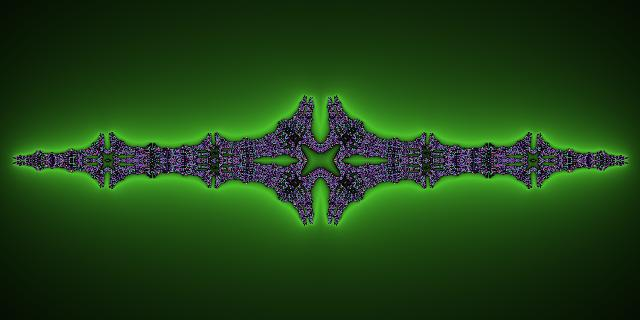c=(-1.15, -0.4)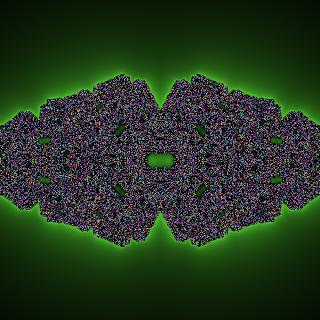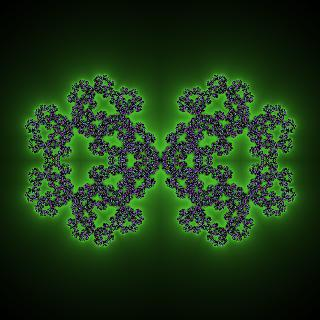c=(-1.15, -0.4) c=(-1, -1)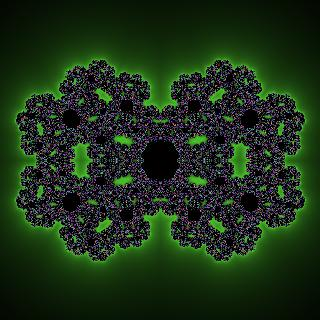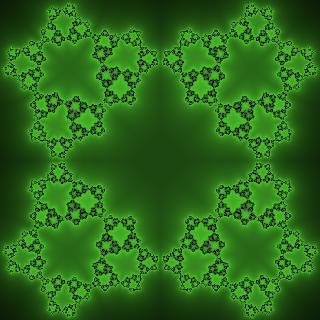c=(-0.75,-0.9) c=(0, -1)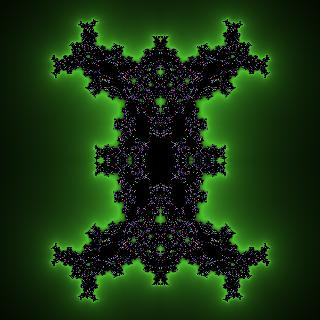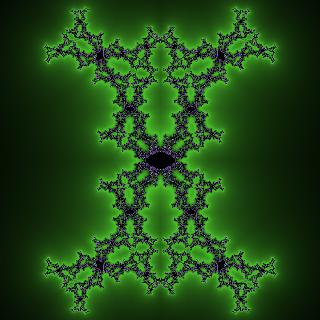c=(0.675, -1.15) c=(0.87,-1.52)c=(0.975, -1.175) c=(0.29, -0.29)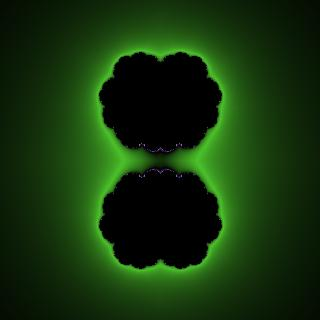c=(0.425, 0.25) c=(0, 0.297)c=(-0.8, 0.1)